Examples

Chapter 2 Class 9 Polynomials (Term 2)
Serial order wise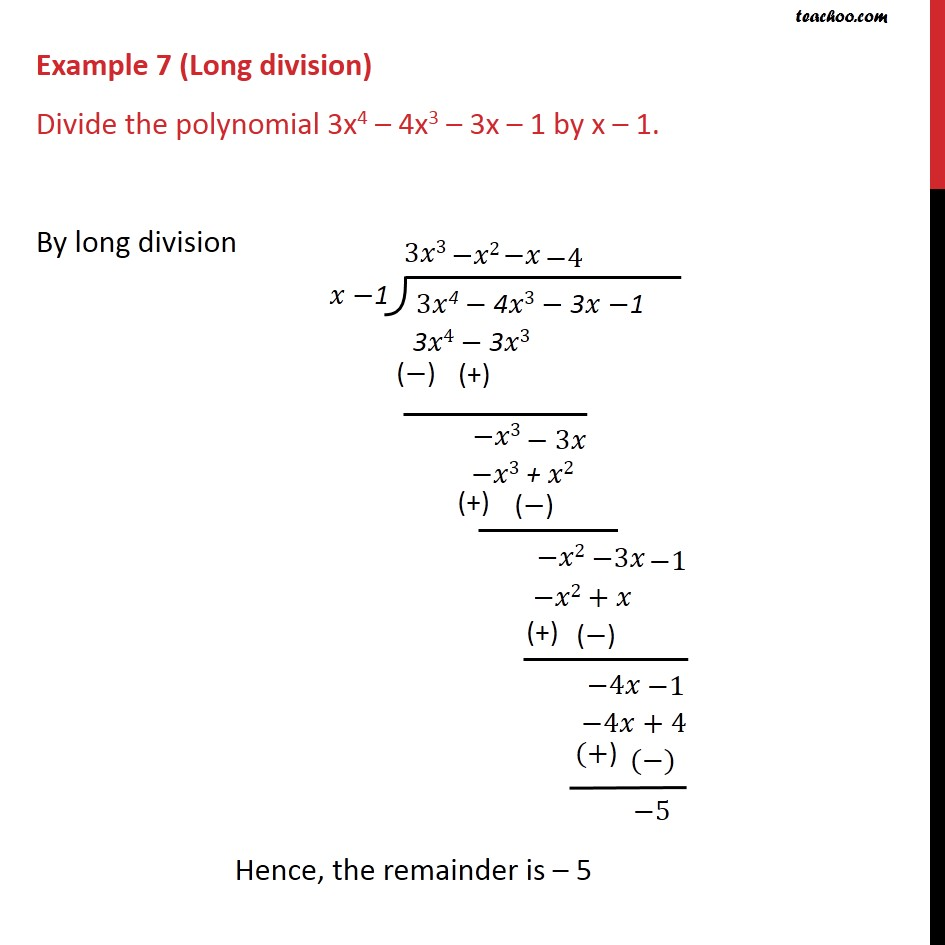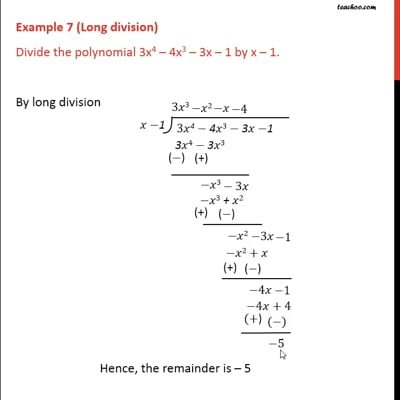This video is only available for Teachoo black users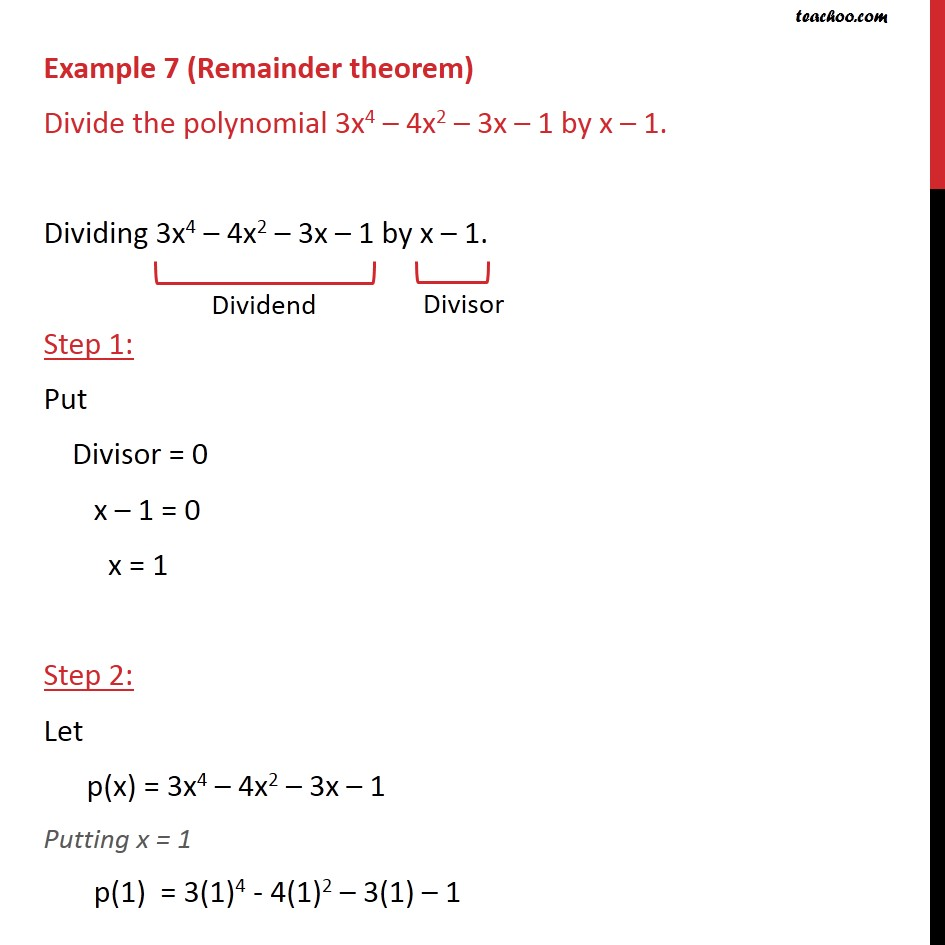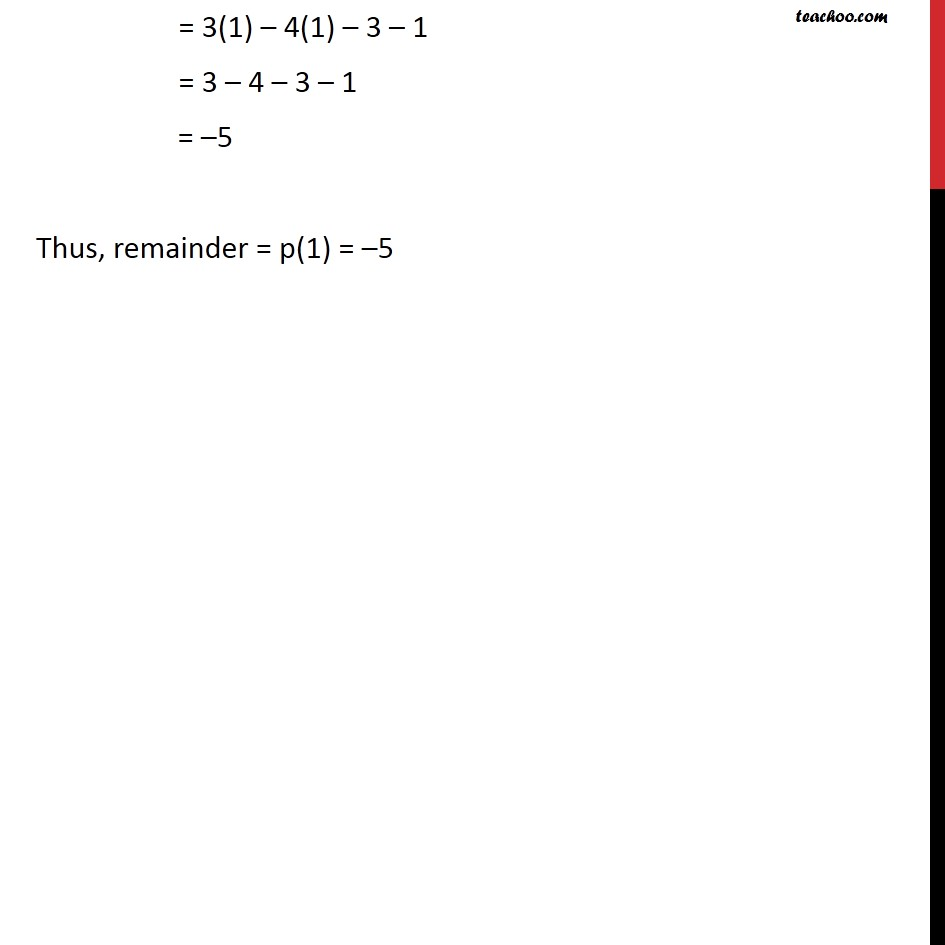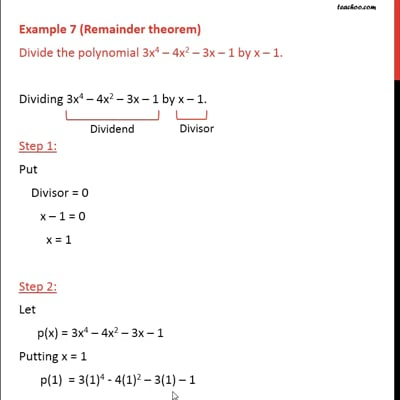This video is only available for Teachoo black users

### Transcript

Example 7 (Long division) Divide the polynomial 3x4 – 4x3 – 3x – 1 by x – 1. By long division Example 7 (Remainder theorem) Divide the polynomial 3x4 – 4x2 – 3x – 1 by x – 1. Dividing 3x4 – 4x2 – 3x – 1 by x – 1. Step 1: Put Divisor = 0 x – 1 = 0 x = 1 Step 2: Let p(x) = 3x4 – 4x2 – 3x – 1 Putting x = 1 p(1) = 3(1)4 - 4(1)2 – 3(1) – 1 = 3(1) – 4(1) – 3 – 1 = 3 – 4 – 3 – 1 = –5 Thus, remainder = p(1) = –5